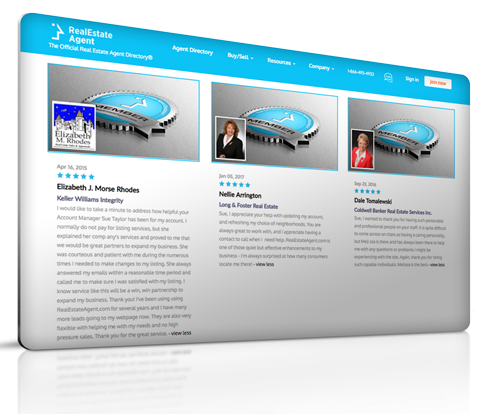# Straight-line Depreciation

## Definition of "Straight-line depreciation"

Danielle Eliot, Real Estate Agent Better Homes & Gardens Metro Brokers

The depreciation method where an equal amount of depreciation expense is allocated to each full period of the asset's useful life. The amount of depreciation is computed as follows; Annual depreciation = (Original costs -Salvage value)/ Useful life. For example, assume that the building costs \$800,000 and has an estimated useful life of 20 years. The estimated salvage value at the end of the 5-year period is \$200,000. Then the straight-line depreciation per year is (\$800,000 - \$200,000)/20 years= \$30,000/year.

#### Have a question or comment?We're here to help.